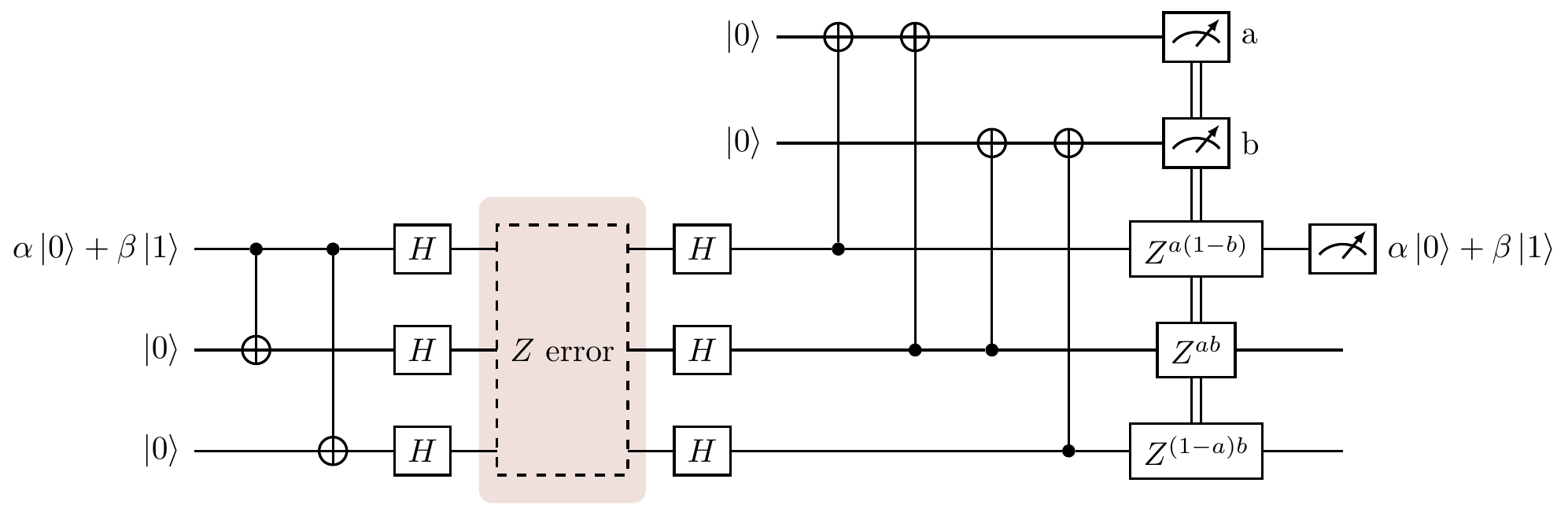## 13.8 Correcting phase-flips

We have seen how the classical [3,1,3]-code can be adapted to detect and correct for a single quantum bit flip, but in Section 13.4 we said that there are three possible errors that we need to worry about: bit-flips, phase-flips, and bit-and-phase flips. Having dealt with the first, we now deal with the second; finding a way to combine these two solutions to deal with the third is the subject of Section 13.10.

It turns out that we really don’t need to do much work in order to solve the problem of single phase-flip errors if we make use of the fact that HZH=X, i.e. phase-flips become bit-flips when sandwiched between Hadamards!Figure 13.9: Using the quantised [3,1,3]-code to deal with phase-flips by sandwiching the transmission area between Hadamards.

The encoded state that enters the transmission area affected by decoherence now reads \alpha|+++\rangle+\beta|---\rangle, where |\pm\rangle=(|0\rangle\pm|1\rangle)/\sqrt{2}. These are eigenstates of Z, i.e. Z|\pm\rangle=|\mp\rangle, and so errors get transformed into orthogonal, and thus detectable, states.

But just as how the circuit in Section 13.7 only protected against bit-flips, this circuit only protects against phase-flips — now we need to find a way to combine them.